Скачать презентацию ETM 607 Statistical Models in Simulation

f63dd2e9e8f9289d518242f381004514.ppt

• Количество слайдов: 29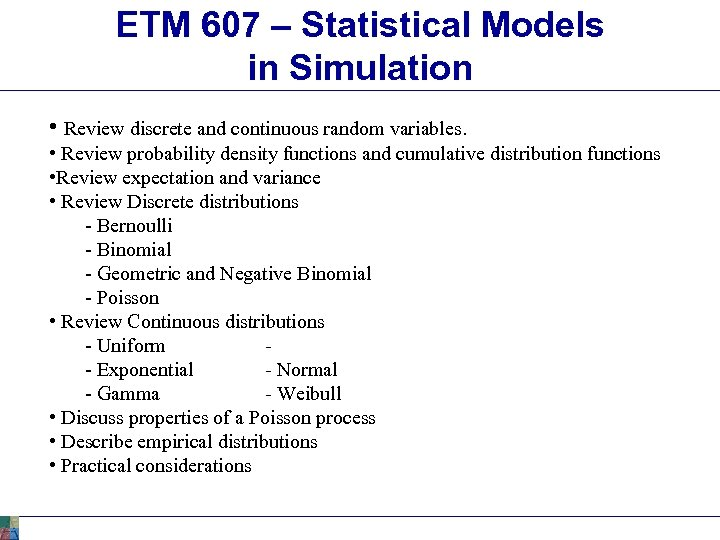ETM 607 – Statistical Models in Simulation • Review discrete and continuous random variables. • Review probability density functions and cumulative distribution functions • Review expectation and variance • Review Discrete distributions - Bernoulli - Binomial - Geometric and Negative Binomial - Poisson • Review Continuous distributions - Uniform - Exponential - Normal - Gamma - Weibull • Discuss properties of a Poisson process • Describe empirical distributions • Practical considerations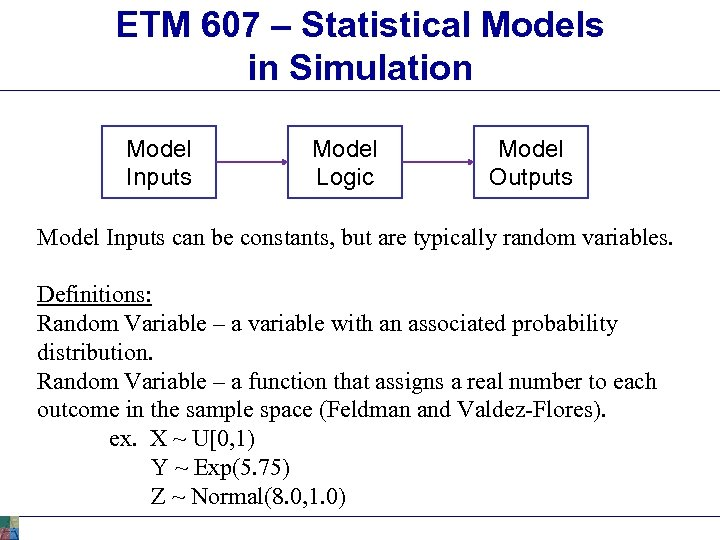ETM 607 – Statistical Models in Simulation Model Inputs Model Logic Model Outputs Model Inputs can be constants, but are typically random variables. Definitions: Random Variable – a variable with an associated probability distribution. Random Variable – a function that assigns a real number to each outcome in the sample space (Feldman and Valdez-Flores). ex. X ~ U[0, 1) Y ~ Exp(5. 75) Z ~ Normal(8. 0, 1. 0)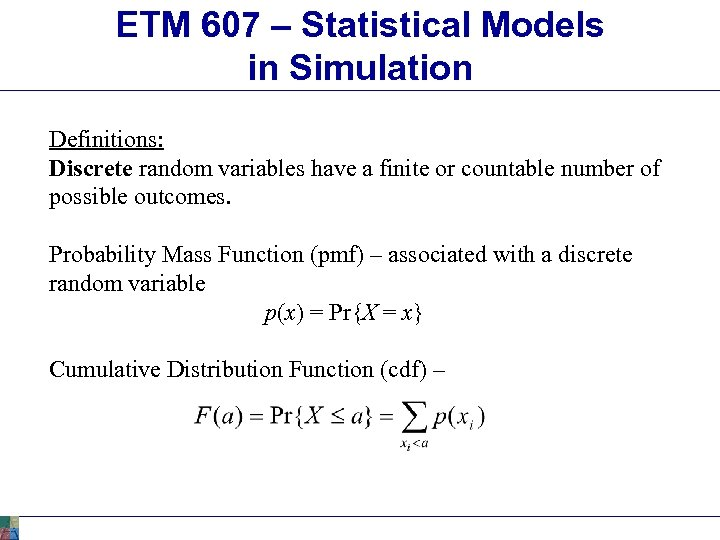ETM 607 – Statistical Models in Simulation Definitions: Discrete random variables have a finite or countable number of possible outcomes. Probability Mass Function (pmf) – associated with a discrete random variable p(x) = Pr{X = x} Cumulative Distribution Function (cdf) –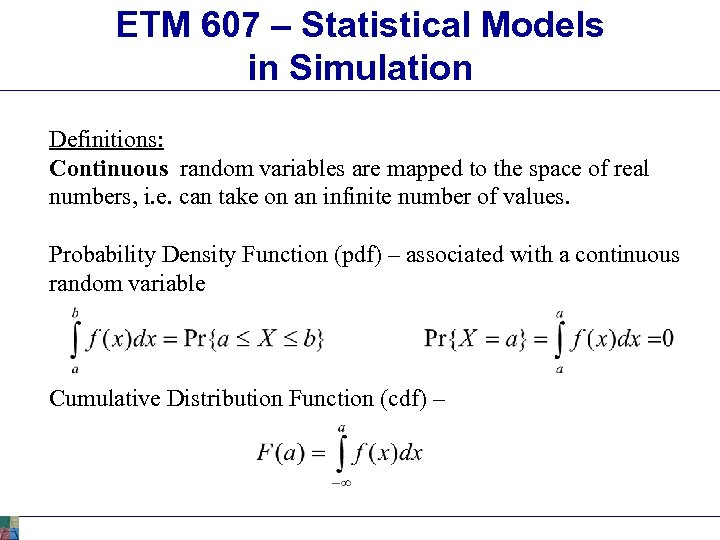ETM 607 – Statistical Models in Simulation Definitions: Continuous random variables are mapped to the space of real numbers, i. e. can take on an infinite number of values. Probability Density Function (pdf) – associated with a continuous random variable Cumulative Distribution Function (cdf) –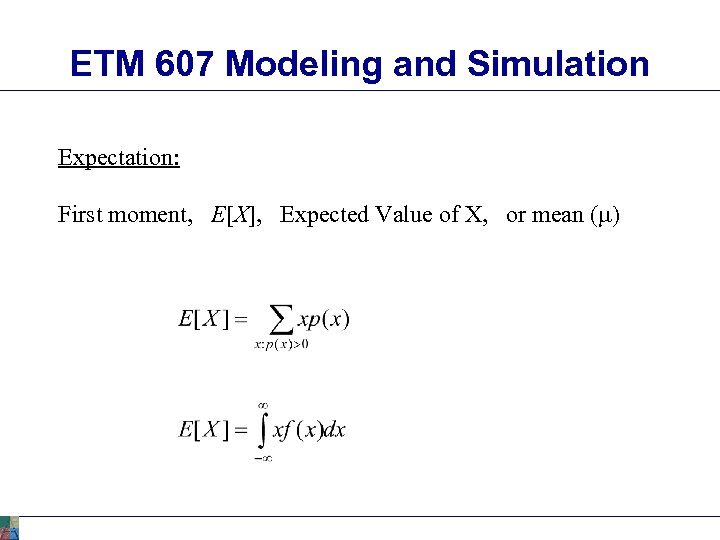ETM 607 Modeling and Simulation Expectation: First moment, E[X], Expected Value of X, or mean (m)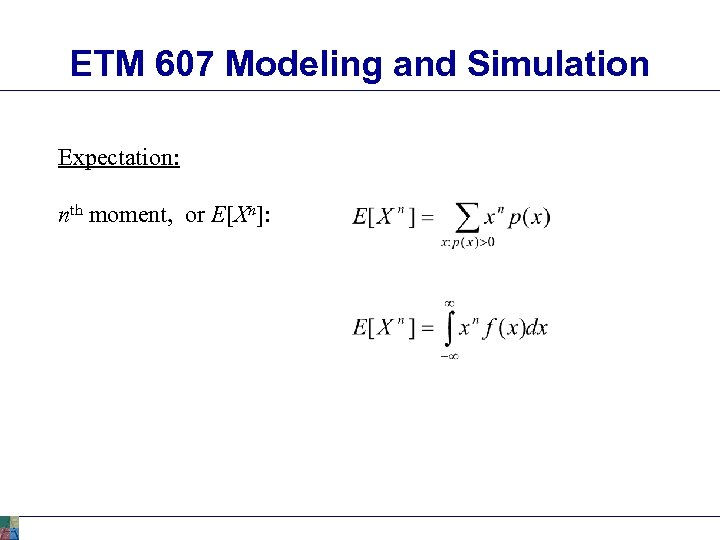ETM 607 Modeling and Simulation Expectation: nth moment, or E[Xn]: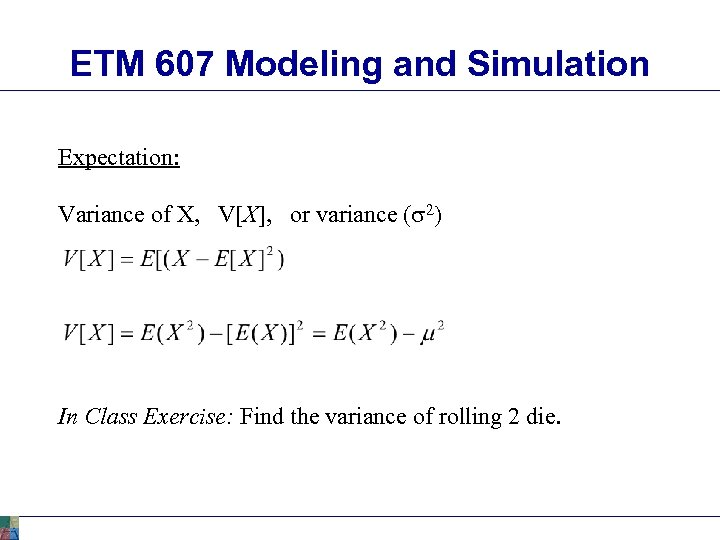ETM 607 Modeling and Simulation Expectation: Variance of X, V[X], or variance (s 2) In Class Exercise: Find the variance of rolling 2 die.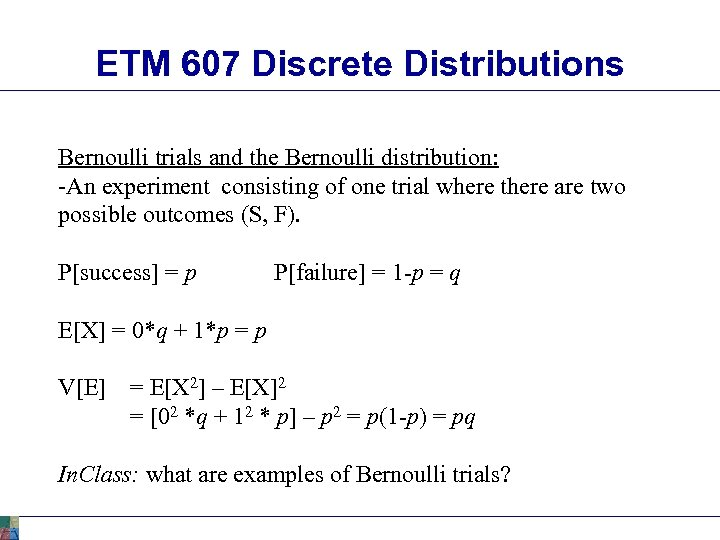ETM 607 Discrete Distributions Bernoulli trials and the Bernoulli distribution: -An experiment consisting of one trial where there are two possible outcomes (S, F). P[success] = p P[failure] = 1 -p = q E[X] = 0*q + 1*p = p V[E] = E[X 2] – E[X]2 = [02 *q + 12 * p] – p 2 = p(1 -p) = pq In. Class: what are examples of Bernoulli trials?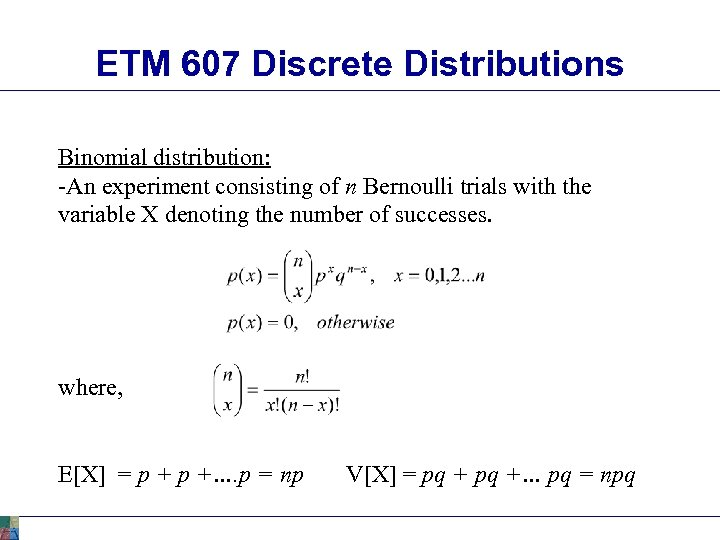ETM 607 Discrete Distributions Binomial distribution: -An experiment consisting of n Bernoulli trials with the variable X denoting the number of successes. where, E[X] = p +…. p = np V[X] = pq +… pq = npq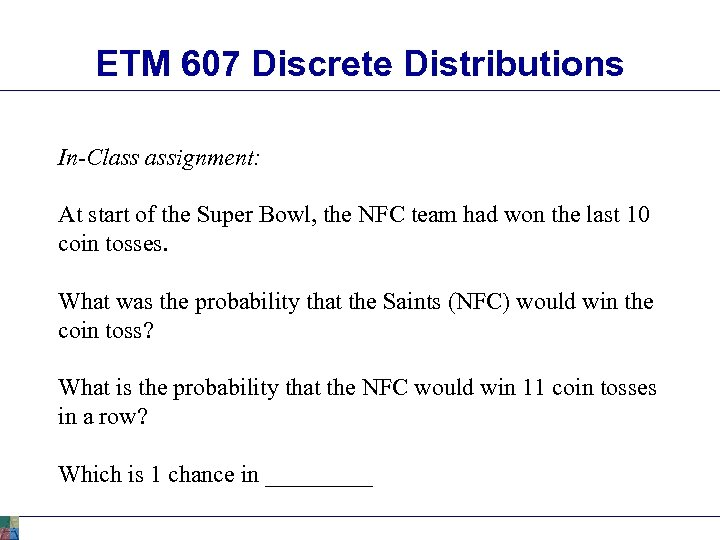ETM 607 Discrete Distributions In-Class assignment: At start of the Super Bowl, the NFC team had won the last 10 coin tosses. What was the probability that the Saints (NFC) would win the coin toss? What is the probability that the NFC would win 11 coin tosses in a row? Which is 1 chance in _____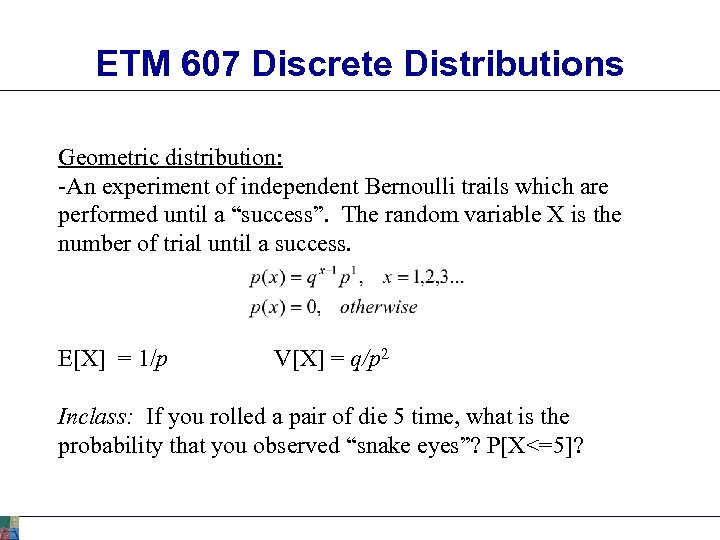ETM 607 Discrete Distributions Geometric distribution: -An experiment of independent Bernoulli trails which are performed until a “success”. The random variable X is the number of trial until a success. E[X] = 1/p V[X] = q/p 2 Inclass: If you rolled a pair of die 5 time, what is the probability that you observed “snake eyes”? P[X<=5]?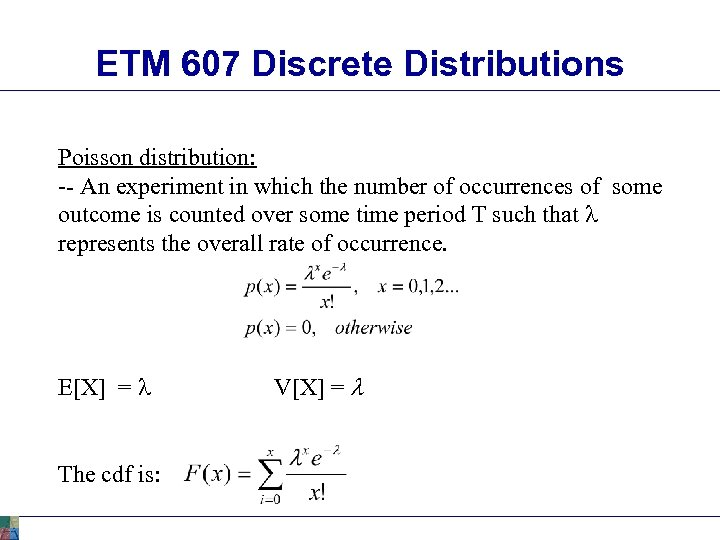ETM 607 Discrete Distributions Poisson distribution: -- An experiment in which the number of occurrences of some outcome is counted over some time period T such that l represents the overall rate of occurrence. E[X] = l The cdf is: V[X] = l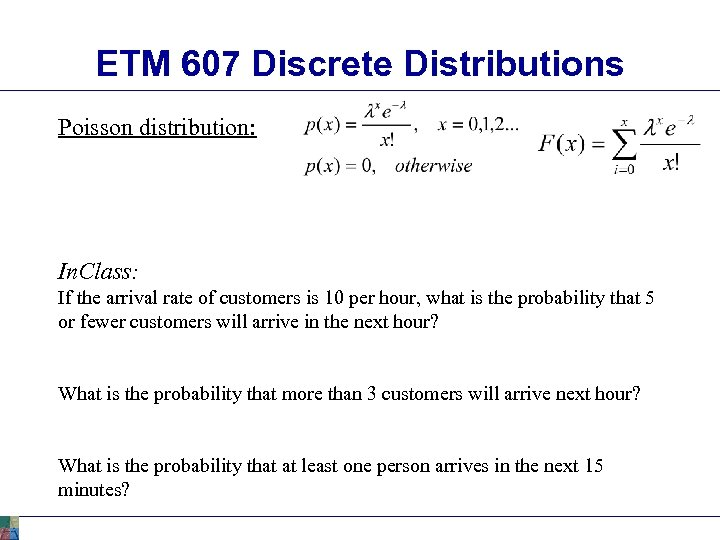ETM 607 Discrete Distributions Poisson distribution: In. Class: If the arrival rate of customers is 10 per hour, what is the probability that 5 or fewer customers will arrive in the next hour? What is the probability that more than 3 customers will arrive next hour? What is the probability that at least one person arrives in the next 15 minutes?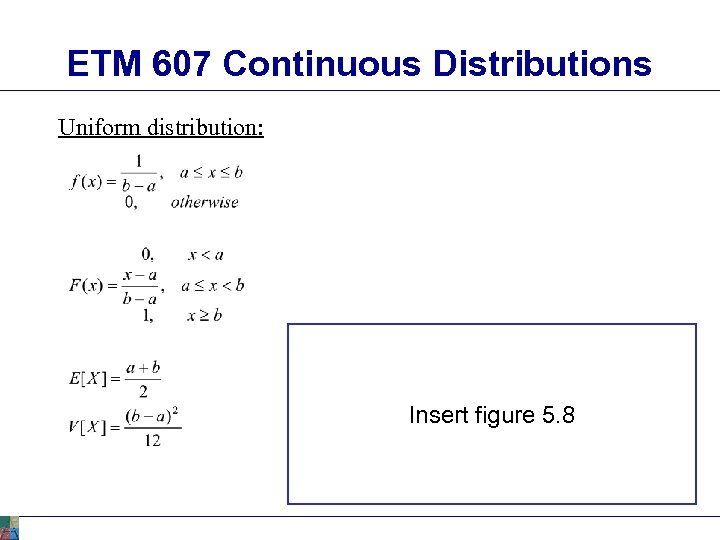ETM 607 Continuous Distributions Uniform distribution: Insert figure 5. 8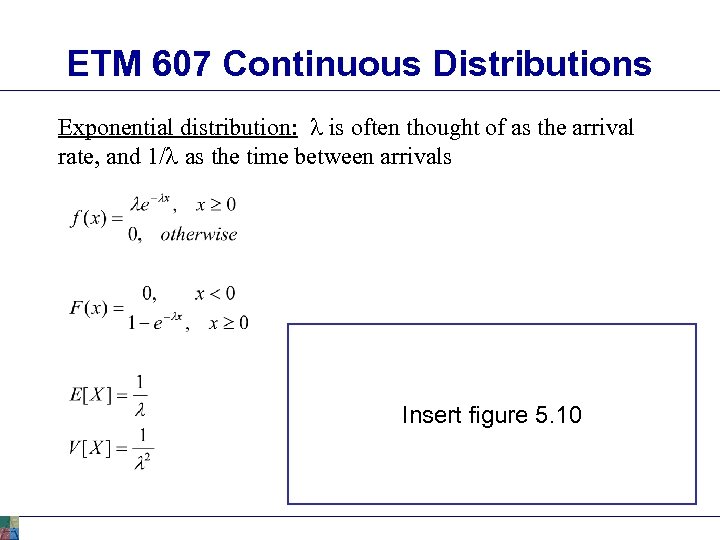ETM 607 Continuous Distributions Exponential distribution: l is often thought of as the arrival rate, and 1/l as the time between arrivals Insert figure 5. 10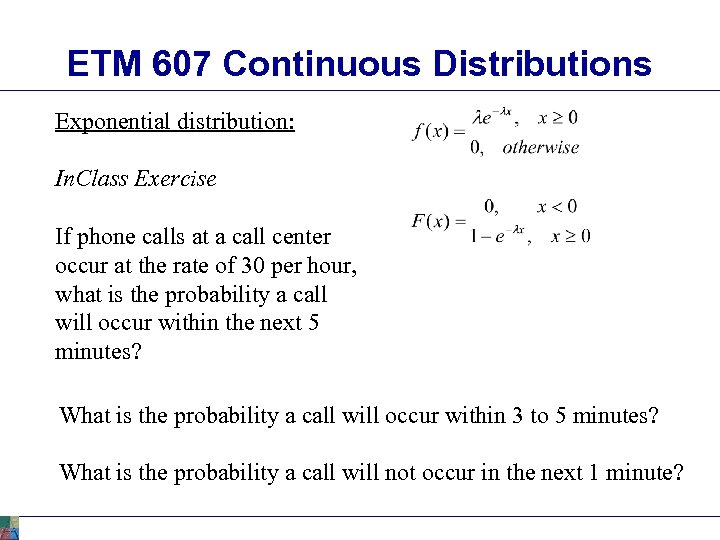ETM 607 Continuous Distributions Exponential distribution: In. Class Exercise If phone calls at a call center occur at the rate of 30 per hour, what is the probability a call will occur within the next 5 minutes? What is the probability a call will occur within 3 to 5 minutes? What is the probability a call will not occur in the next 1 minute?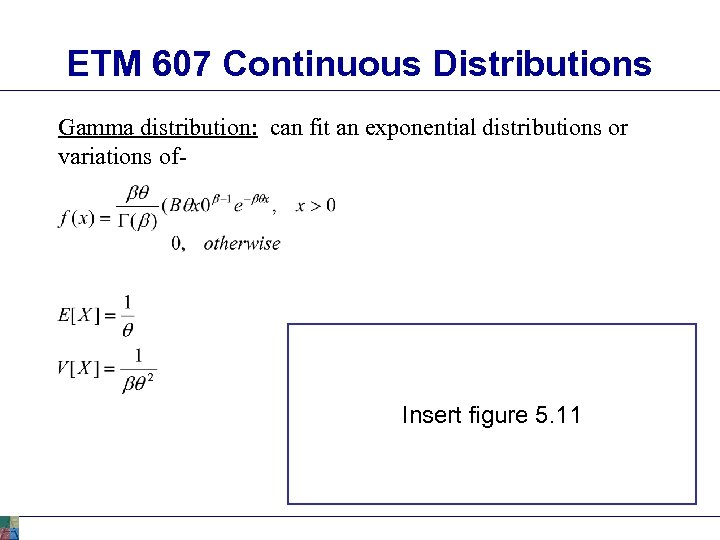ETM 607 Continuous Distributions Gamma distribution: can fit an exponential distributions or variations of- Insert figure 5. 11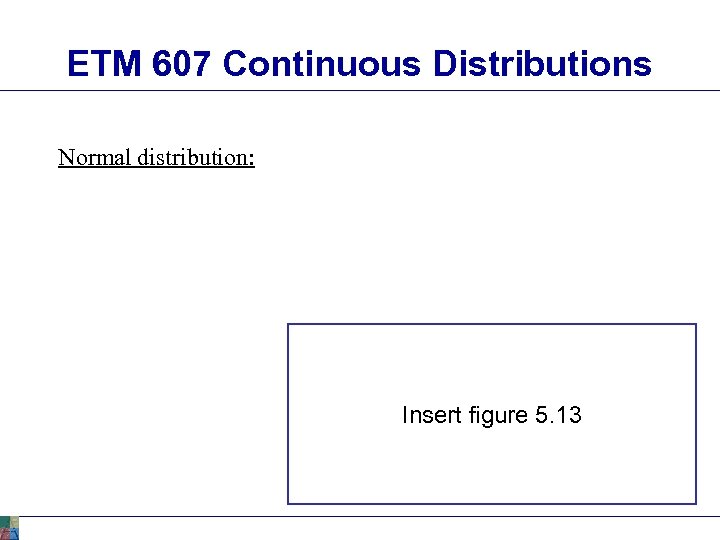ETM 607 Continuous Distributions Normal distribution: Insert figure 5. 13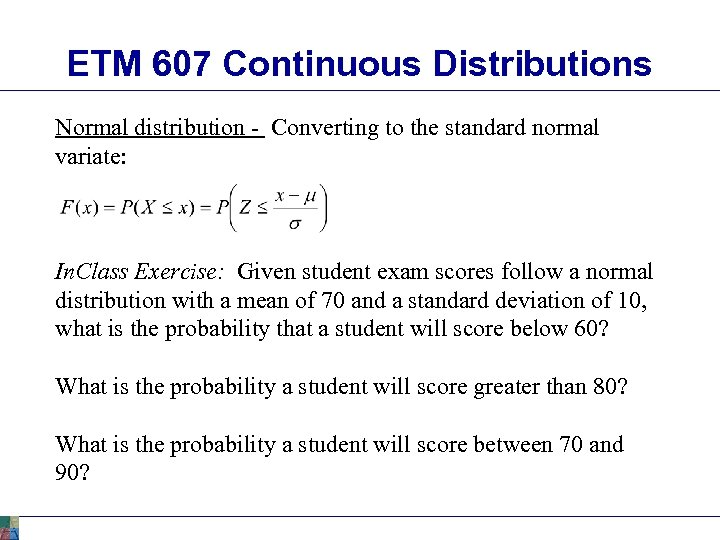ETM 607 Continuous Distributions Normal distribution - Converting to the standard normal variate: In. Class Exercise: Given student exam scores follow a normal distribution with a mean of 70 and a standard deviation of 10, what is the probability that a student will score below 60? What is the probability a student will score greater than 80? What is the probability a student will score between 70 and 90?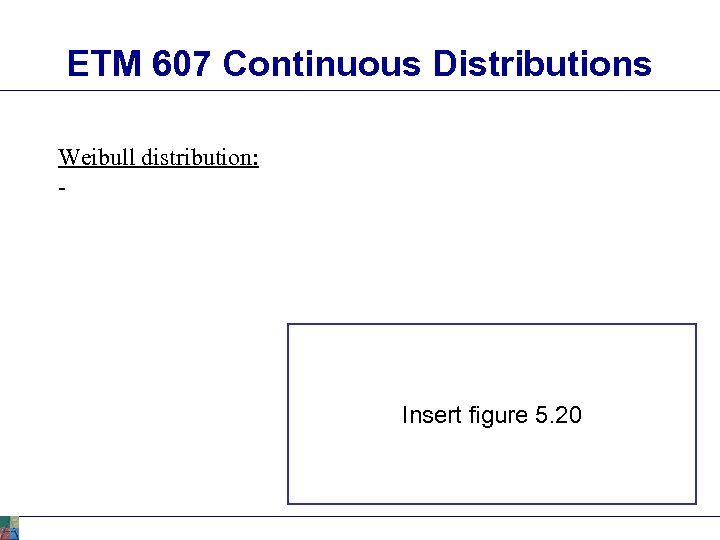ETM 607 Continuous Distributions Weibull distribution: - Insert figure 5. 20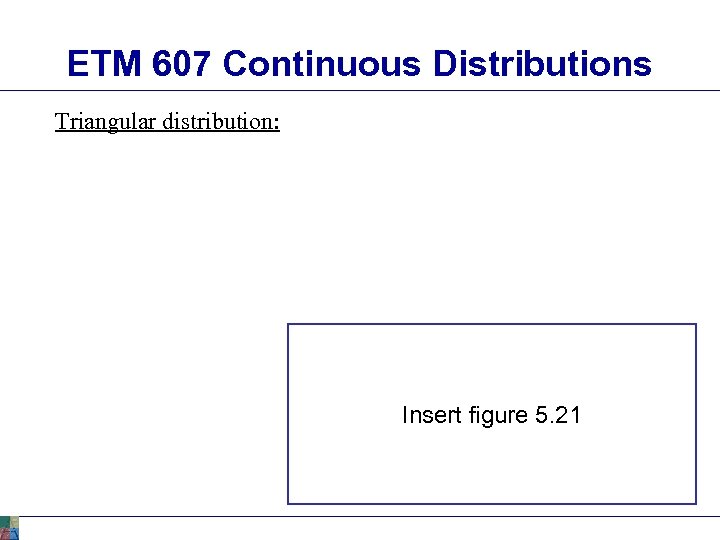ETM 607 Continuous Distributions Triangular distribution: Insert figure 5. 21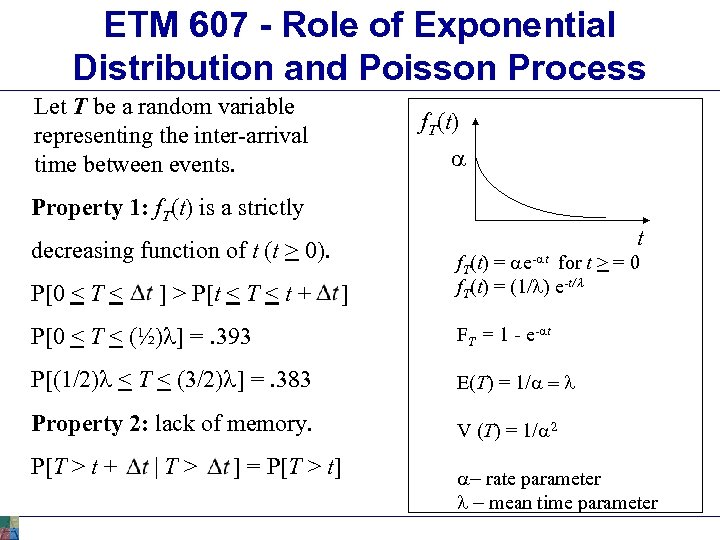ETM 607 - Role of Exponential Distribution and Poisson Process Let T be a random variable representing the inter-arrival time between events. f. T(t) a Property 1: f. T(t) is a strictly t decreasing function of t (t > 0). P[0 < T < ] > P[t < T < t + ] f. T(t) = ae-at for t > = 0 f. T(t) = (1/l) e-t/l P[0 < T < (½)l] =. 393 FT = 1 - e-at P[(1/2)l < T < (3/2)l] =. 383 E(T) = 1/a = l Property 2: lack of memory. V (T) = 1/a 2 P[T > t + |T> ] = P[T > t] a- rate parameter l - mean time parameter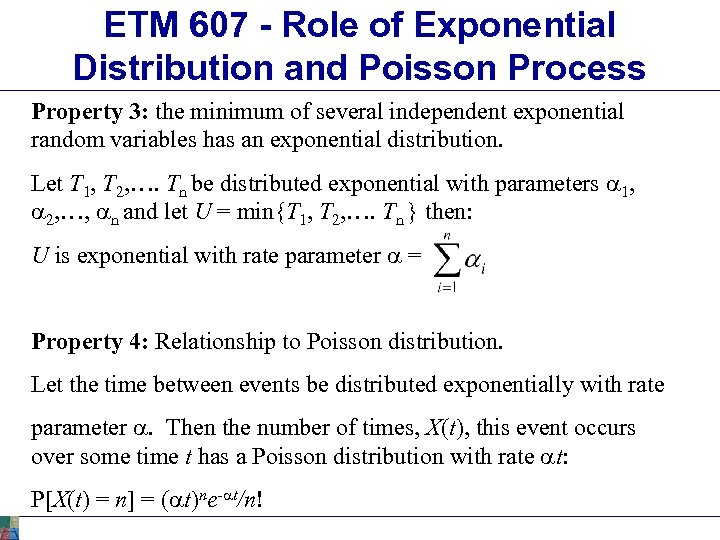ETM 607 - Role of Exponential Distribution and Poisson Process Property 3: the minimum of several independent exponential random variables has an exponential distribution. Let T 1, T 2, …. Tn be distributed exponential with parameters a 1, a 2, …, an and let U = min{T 1, T 2, …. Tn } then: U is exponential with rate parameter a = Property 4: Relationship to Poisson distribution. Let the time between events be distributed exponentially with rate parameter a. Then the number of times, X(t), this event occurs over some time t has a Poisson distribution with rate at: P[X(t) = n] = (at)ne-at/n!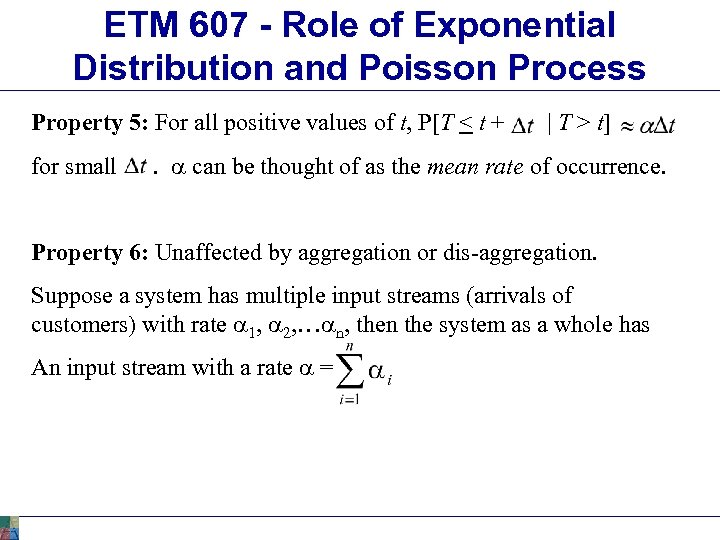ETM 607 - Role of Exponential Distribution and Poisson Process Property 5: For all positive values of t, P[T < t + for small | T > t] . a can be thought of as the mean rate of occurrence. Property 6: Unaffected by aggregation or dis-aggregation. Suppose a system has multiple input streams (arrivals of customers) with rate a 1, a 2, …an, then the system as a whole has An input stream with a rate a =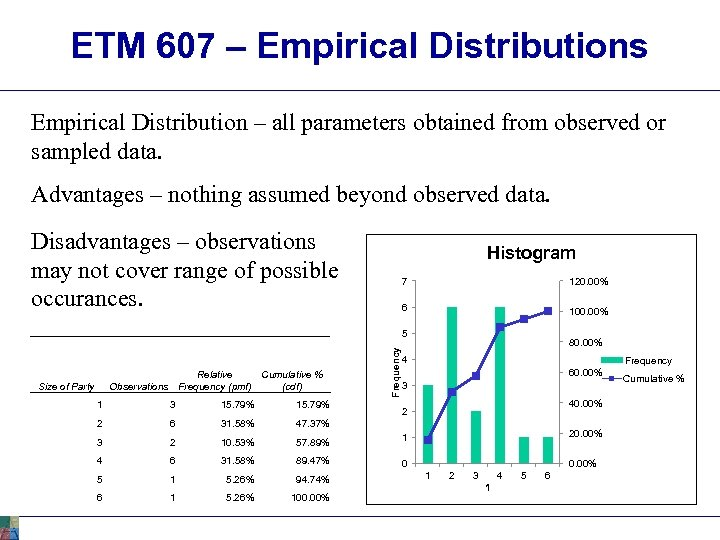ETM 607 – Empirical Distributions Empirical Distribution – all parameters obtained from observed or sampled data. Advantages – nothing assumed beyond observed data. Disadvantages – observations may not cover range of possible occurances. Histogram 7 120. 00% 6 100. 00% Relative Cumulative % Observations Frequency (pmf) (cdf) Size of Party Frequency 5 80. 00% 4 Frequency 60. 00% 3 15. 79% 2 6 31. 58% 47. 37% 3 2 10. 53% 57. 89% 1 4 6 31. 58% 89. 47% 40. 00% 0 5 1 5. 26% 94. 74% 6 1 5. 26% 100. 00% 2 20. 00% 1 2 3 4 1 5 6 Cumulative %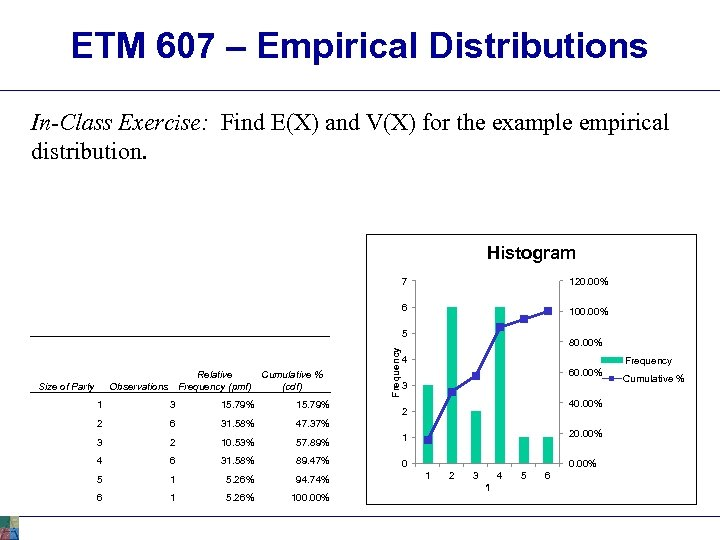ETM 607 – Empirical Distributions In-Class Exercise: Find E(X) and V(X) for the example empirical distribution. Histogram 7 120. 00% 6 100. 00% Relative Cumulative % Observations Frequency (pmf) (cdf) Size of Party Frequency 5 80. 00% 4 Frequency 60. 00% 3 15. 79% 2 6 31. 58% 47. 37% 3 2 10. 53% 57. 89% 1 4 6 31. 58% 89. 47% 40. 00% 0 5 1 5. 26% 94. 74% 6 1 5. 26% 100. 00% 2 20. 00% 1 2 3 4 1 5 6 Cumulative %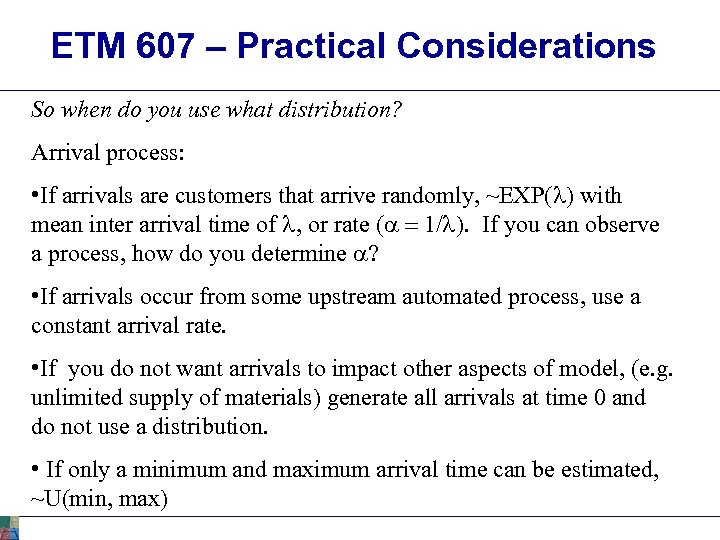ETM 607 – Practical Considerations So when do you use what distribution? Arrival process: • If arrivals are customers that arrive randomly, ~EXP(l) with mean inter arrival time of l, or rate (a = 1/l). If you can observe a process, how do you determine a? • If arrivals occur from some upstream automated process, use a constant arrival rate. • If you do not want arrivals to impact other aspects of model, (e. g. unlimited supply of materials) generate all arrivals at time 0 and do not use a distribution. • If only a minimum and maximum arrival time can be estimated, ~U(min, max)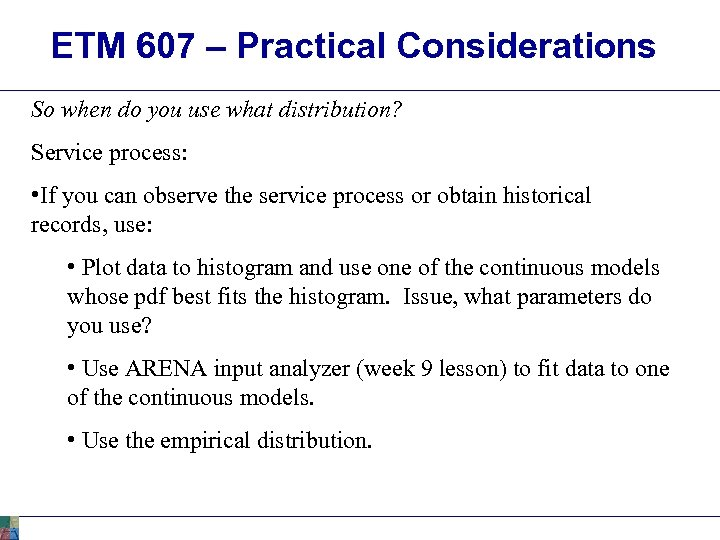ETM 607 – Practical Considerations So when do you use what distribution? Service process: • If you can observe the service process or obtain historical records, use: • Plot data to histogram and use one of the continuous models whose pdf best fits the histogram. Issue, what parameters do you use? • Use ARENA input analyzer (week 9 lesson) to fit data to one of the continuous models. • Use the empirical distribution.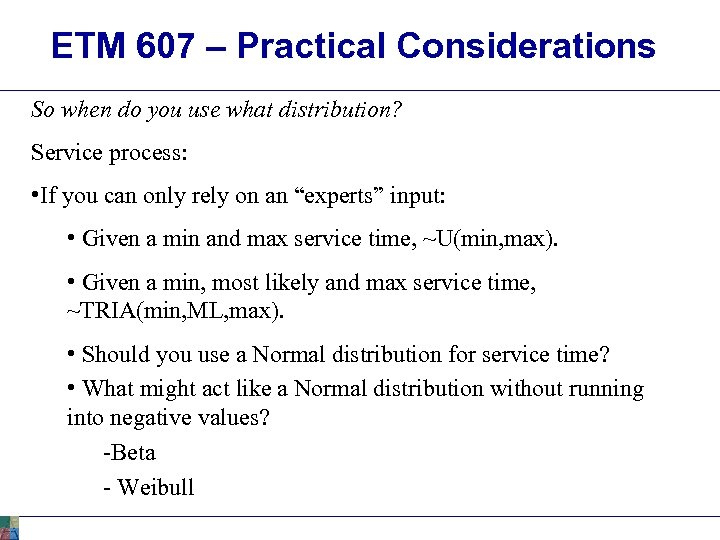ETM 607 – Practical Considerations So when do you use what distribution? Service process: • If you can only rely on an “experts” input: • Given a min and max service time, ~U(min, max). • Given a min, most likely and max service time, ~TRIA(min, ML, max). • Should you use a Normal distribution for service time? • What might act like a Normal distribution without running into negative values? -Beta - Weibull